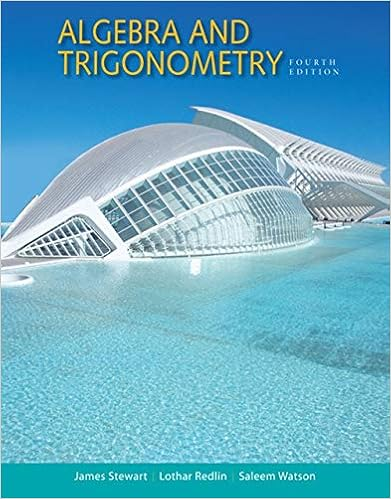# 324.midterm02.2005 - STAT-324 Midterm II April 5 2005...

• Notes
• 5

This preview shows page 1 - 5 out of 5 pages.

##### We have textbook solutions for you!
The document you are viewing contains questions related to this textbook.The document you are viewing contains questions related to this textbook.
Chapter 14 / Exercise 40
Algebra and Trigonometry
Redlin/StewartExpert Verified
STAT-324Midterm IIApril 5, 20059:30-10:40AMInstructor: Moo K. ChungAnswer all questions showing all your workneatly.Calculators are allowed. One page note is permittedName:Student ID:Pledge: On my honor, I have neither given nor receivedunauthorized aid on this examination.Signature:QuestionPoints1234Total
##### We have textbook solutions for you!
The document you are viewing contains questions related to this textbook.The document you are viewing contains questions related to this textbook.
Chapter 14 / Exercise 40
Algebra and Trigonometry
Redlin/StewartExpert Verified
1. A box contains 7 white balls and 3 black balls. A ball is chosen at random and replaced by theopposite color. Now again draw a ball.(a) What is the probability that the second ball drawn is white? [5pts](b) What is the probability that the first ball drawn is black when the second ball drawn iswhite? [5pts]
2. The joint distribution ofXandYis given byf(x, y)=2 fory < x <1,0< y <10 otherwise.(a) Compute the probabilityP(12< X <1,12< Y <1). [5pts](b) Determine the marginal distributions ofXandY. [5pts]
•••